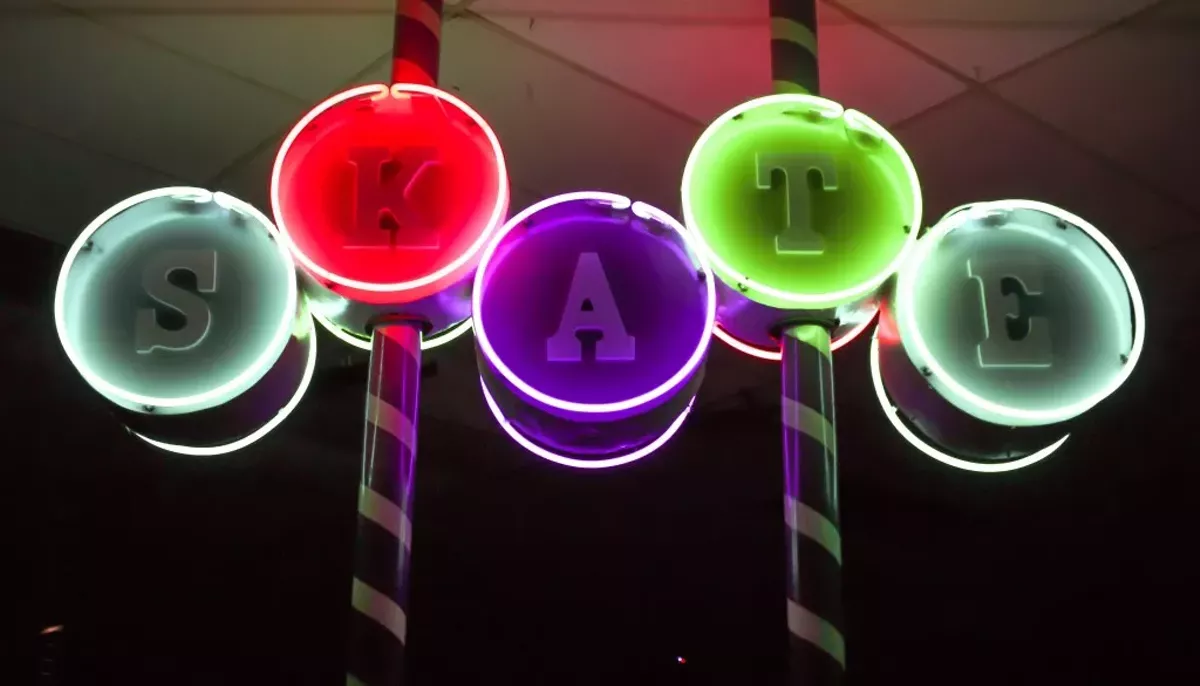# 43 Photos of Boogie Night at The Rollercade

Tweet
Submit to Reddit
Email
Leave it to The Rollercade to throw the grooviest disco party of the summer.
Photos by Julian P. Ledezma
OF 43
PREV NEXTJulian P. Ledezma
Julian P. Ledezma
Julian P. Ledezma
Julian P. Ledezma
Julian P. Ledezma
Julian P. Ledezma
Julian P. Ledezma
Julian P. Ledezma
Julian P. Ledezma
Julian P. Ledezma
Julian P. Ledezma
Julian P. Ledezma
Julian P. Ledezma
Julian P. Ledezma
Julian P. Ledezma
Julian P. Ledezma
Julian P. Ledezma
Julian P. Ledezma
Julian P. Ledezma
Julian P. Ledezma
Julian P. Ledezma
Julian P. Ledezma
Julian P. Ledezma
Julian P. Ledezma
Julian P. Ledezma
Julian P. Ledezma
Julian P. Ledezma
Julian P. Ledezma
Julian P. Ledezma
Julian P. Ledezma
Julian P. Ledezma
Julian P. Ledezma
Julian P. Ledezma
Julian P. Ledezma
Julian P. Ledezma
Julian P. Ledezma
Julian P. Ledezma
Julian P. Ledezma
Julian P. Ledezma
Julian P. Ledezma